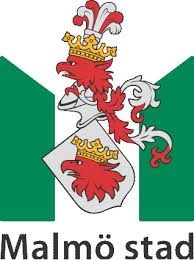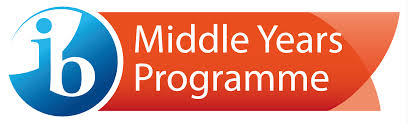Subject Sites‎ > ‎

### Mathematics

Mathematics has been divided into two groups;

-> Standard maths
-> Extended maths

• Hi MYP,

->   If you're interested to do more maths, I would recommend you to visit                     kutasoftware.com which has free worksheets.

Click:
-> Kutasoftware.
-> Web Maths

Assessed units
MYP4                                 MYP3
Unit:  Statistics and probability

Content: Chap. 15 & 16
Main topics:
• Mean, median, mode
• Frequency graphs/ tables
• Standard deviation
• Event and the chance
• Venn diagram, tree diagram
• Dependent / independent events

### MYP assessment criteria:

• A: Knowing and understanding
• B: Investigating patterns
• C: Communicating
• D: Applying mathematics in real-life contexts
Test Date: 2014.12.02
Unit: Algebraic expressions, equations and inequalities

Content: Chap. 4,6 & 7
Main topics:
• Operations on algebraic expressions
• Operation on index
• Algebraic equations
• Algebraic inequalities

### MYP assessment criteria:

• A: Knowing and understanding
• B: Investigating patterns
• C: Communicating
• D: Applying mathematics in real-life contexts

Test Date: 2014.12.01

Neglect of mathematics works injury to all knowledge, since he who is ignorant of it cannot know the other sciences or the things of the world.     Roger Bacon

Mathematics is a fun land which contains bunches of trees. Near the beach,it's a nice wooden place on the water."

Current units
 MYP1 MYP2 MYP3 MYP4 Percentages, ratios and rates Working mathematically Equations and graphs

Pythagorean theorem

http://www.regentsprep.org/regents/math/algebra/AT1/PracPyth.htmĊ
Nov 25, 2014, 5:34 AM
Ċ
Nov 21, 2014, 2:01 AM
Ċ
Nov 21, 2014, 2:02 AM
Ċ
Jan 12, 2015, 12:50 AM
Ċ